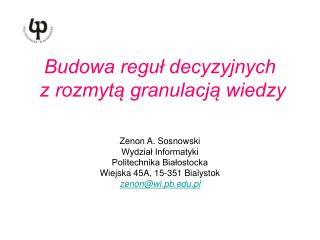DownloadDownload PresentationBudowa reguł decyzyjnych z rozmytą granulacją wiedzy

# Budowa reguł decyzyjnych z rozmytą granulacją wiedzy

Télécharger la présentation## Budowa reguł decyzyjnych z rozmytą granulacją wiedzy

- - - - - - - - - - - - - - - - - - - - - - - - - - - E N D - - - - - - - - - - - - - - - - - - - - - - - - - - -
##### Presentation Transcript

1. Budowa reguł decyzyjnych z rozmytą granulacją wiedzy Zenon A. Sosnowski Wydział Informatyki Politechnika Białostocka Wiejska 45A, 15-351 Bialystok zenon@wi.pb.edu.pl

2. Agenda • wprowadzenie • drzewa decyzyjne (DT) • zbiory rozmyte w granulacji atrybutów • algorytm generowania kontekstowych DT • przykład • wnioski

3. Rozmyta sieć RETE The inference mechanism realizes a generalized modus ponens rule. if A then C CFr A'CFf ---------------------- C' CFc CFr is an uncertainty of the rule CFf is an uncertainty of the fact CFc is an uncertainty of the conclusion CFc = CFr * CFf

4. Fuzzy_Fuzzy

5. (speed medium) - WME SINGLE (LV speed) MULTIFIELD End of pattern M.(very fast) (attached) M.(slow) (attached) activation rule r2 (defrule r1(speed very fast)=> ( . . . )) (defrule r2(speed slow)=> ( . . . ))

6. Decicion Trees – An Overview • used to solve classification problems • structure of problem - attributes - each attribute assumes a finite number values - finite number of discrete classes • entropy-based optimization criterion • architecture of decision tree: nodes – attributes, edges – values of attributes

7. Coping with Continuous Attributes Decision trees require finite-valued attributes What if attributes are continuous ? Attributes need to be discretrized Options: - discretize each attribute separately (uniform and nonuniform) - discretize all attributes (clustering)

8. Quantization of attributes through clustering • Fuzzy Clustering • Context-based fuzzy clustering

9. Fuzzy Clustering (FCM) versus Context-Based FCM (cFCM) Fuzzy clustering: objective function and its iteraive optimization Context-base fuzzy clustering: - objective function minimized iteratively - continuous classification variable granulated with the use of linguistic labels

10. Context-Based Fuzzy Clustering Given: data {xk,yk}, k=1,2,…,N, number of clusters (c), distance function ||.||, fuzzy set of context A defined over yk Constrained-based optimization of objective function subject to

11. Context-Based Fuzzy Clustering:An Iterative Optimization Process Given: The number of clusters (c). Select the distance function ||.||, termination criterion e (>0) and initialize partition matrix U U. Select the value of the fuzzification parameter “m” (the default is m=2.0) • Calculate centers (prototypes) of the clusters i=1, 2, ..., c 2. Update partition matrix i=1, 2, ..., c, j=1, 2, ..., N 3. Compare U' to U, if termination criterion ||U’ - U|| <e is satisfied then stop, else return to step (1) and proceed with computing by setting up U equal to U' Result: partition matrix and prototypes

12. Information Granules in the Development of Decision Trees • define contexts (fuzzy sets) for continuous classivication variable • cluster data for each context • project prototypes on the individual axes – this leads to their discretization • carry out the standard ID-3 algorithm W. Pedrycz, Z.A. Sosnowski, „The designing of decision trees in the framework of granular data and their application to software quality models”, Fuzzy Sets & Sysytems, vol. 124, (2001), p. 271-290

13. Fuzzy Sets of Contexts: Two Approaches • subjective selection depending on the classification problem • supported by statistical relevance (σ-count of fuzzy contexts)

14. Constructing linguistic terms – classes (thin line) and their induced interval-valued counterparts (solid line)

15. C - Fuzzy Decision Trees W. Pedrycz, Z.A. Sosnowski, „C-Fuzzy Decision Trees”, IEEE Transactions on Systems, Man and Cybernetics, Part C, Vol. 35, No 4, 2005, p. 498-511.

16. Architecture of the cluster-based decision tree • cluster all data set X • repeat • allocate elements of X to each cluster • choose the node with the highest value of the spliting criterion • cluster data at selected node untiltermination criterion is fulfield

17. Node splitting criterion Node of the tree Ni = <Xi, Yi, Ui> where: Xi = { x(k) | ui(x(k)) > uj(x(k))} Yi = {y(k)| x(k)εXi} Ui = [ui(x(1)) ui(x(2)) … ui(x(N))]

18. Stopping criterion(structurability index)

19. C-fuzzy tree in the classification (prediction) mode assign x to class wi if ui(x) exceeds the values of the membership in all remaining clusters

20. Experiments Data sets from the UCI repository of Machine Learning Databases (http://www.ics.uci.edu) • Auto-Mpg • Pima-diabetes • Ionosphere • Hepatitis • Dermatology

21. Hepatitis data

22. Dermatology data

23. Architecture of the Context-based Fuzzy Clustered-oriented Decision Tree define contexts (fuzzy sets) for classivication variable for each context do • cluster (cFCM) Xi(data set of i-th context) • repeat • allocate elements of Xi to each cluster • choose the node with the highest value of the spliting criterion • cluster (cFCM) data at selected node until termination criterion is fulfield enddo

24. Problem Implementation issues: • high complexity –> grid or cluster computing • agregation -> testing of different appraches

25. Dziękuję za uwagę